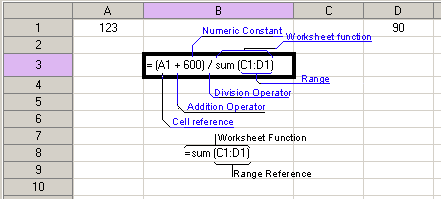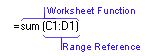# What is a Formula

A formula is an equation that carries out specific operations against worksheet data.  Formulas can perform mathematical operations, such as addition and multiplication, or they can compare worksheet values or join text.  Formulas can refer to other cells on the same worksheet, cells on other sheets in the same workbook.

Note: formulas cannot refer to other cells on sheets in other workbooks.

The following example adds 600 to the value in cell A1 and then divides the result by the sum of the values in cells C1 and E1.Constructing a formula

Formulas calculate values in a specific order.  A formula in the Spreadsheet always begins with an equal sign (=).  An equal sign tells the Spreadsheet that all subsequent characters constitute a formula.  Following the equal sign are the elements to be calculated (the operands), which are separated by calculation operators.  Each element can be a function call with parameters.  The Spreadsheet calculates formulas from left to right, according to the specific order of each operator in the formula.  You can change the order of operations by using parentheses.

Referring to cells

A formula can refer to constant values and to other cells.  A cell that contains a formula is known as a dependent cell when its value depends on other cell values.  For example, cell B2 is a dependent cell if it contains formula "= C2".  There are two types of cell addressing in the Spreadsheet: relative addressing and absolute addressing.  The first assumes that if you delete a column, all addresses will be changed accordingly, its designation is "= C2".  So, if column A is deleted, this address will change to "= B2".

Absolute addressing does not change when a column is deleted, its designation is "= \$C\$2".

Whenever the cell to which a formula refers changes, the dependent cell also changes by default.  If you enter a formula manually, the Auto Recalculation property must be enabled in order to enable automatic formula calculation.

If you use constant values instead of references to cells (for example, "= 30 + 70 + 110"), the result changes only if you modify your formula.

Formulas can also refer to a range of cells.

Using worksheet functions

The Spreadsheet contains a number of predefined or built-in formulas which are known as functions.  Functions can be used to perform simple or complex calculations.  The most frequently used function is the SUM function, which is used to add numbers in a range of cells.

The image above shows how to use the SUM function to add values in range C1:D1.Related Topics